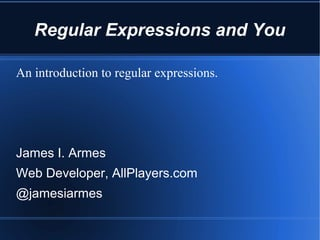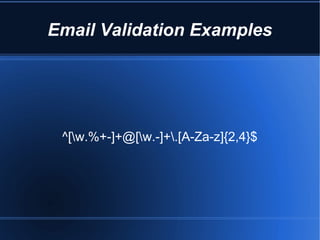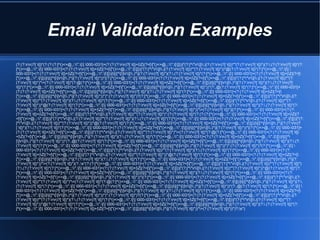Successfully reported this slideshow.

# Regular Expressions and You

0

Share×
1 of 34
1 of 34

# Regular Expressions and You

0

Share

#### Transcript

1. 1. Regular Expressions and You An introduction to regular expressions. James I. Armes Web Developer, AllPlayers.com @jamesiarmes
2. 2. Email Validation Examples ^[w.%+-]+@[w.-]+.[A-Za-z]{2,4}\$
3. 3. Email Validation Examples (?:(?:rn)?[ t])*(?:(?:(?:[^()<>@,;:".[] 000-031]+(?:(?:(?:rn)?[ t])+|Z|(?=[["()<>@,;:".[]]))|"(?:[^"r]|.|(?:(?:rn)?[ t]))*"(?:(?:rn)?[ t])*)(?:.(?:(?:rn)?[ t])*(?: [^()<>@,;:".[] 000-031]+(?:(?:(?:rn)?[ t])+|Z|(?=[["()<>@,;:".[]]))|"(?:[^"r]|.|(?:(?:rn)?[ t]))*"(?:(?:rn)?[ t])*))*@(?:(?:rn)?[ t])*(?:[^()<>@,;:".[] 000-031]+(?:(?:(?:rn)?[ t])+|Z|(?=[["()<>@,;:".[]]))|[([^[]r]|.)*](?:(?:rn)?[ t])*)(?:.(?:(?:rn)?[ t])*(?:[^()<>@,;:".[] 000-031]+(?:(?:(?:rn)?[ t])+|Z|(?=[ ["()<>@,;:".[]]))|[([^[]r]|.)*](?:(?:rn)?[ t])*))*|(?:[^()<>@,;:".[] 000-031]+(?:(?:(?:rn)?[ t])+|Z|(?=[["()<>@,;:".[]]))|"(?:[^"r]|.|(?:(?:rn)?[ t]))*"(?: (?:rn)?[ t])*)*<(?:(?:rn)?[ t])*(?:@(?:[^()<>@,;:".[] 000-031]+(?:(?:(?:rn)?[ t])+|Z|(?=[["()<>@,;:".[]]))|[([^[]r]|.)*](?:(?:rn)?[ t])*)(?:.(?:(?:rn)?[ t])*(?:[^()<>@,;:".[] 000-031]+(?:(?:(?:rn)?[ t])+|Z|(?=[["()<>@,;:".[]]))|[([^[]r]|.)*](?:(?:rn)?[ t])*))*(?:,@(?:(?:rn)?[ t])*(?:[^()<>@,;:".[] 000-031]+ (?:(?:(?:rn)?[ t])+|Z|(?=[["()<>@,;:".[]]))|[([^[]r]|.)*](?:(?:rn)?[ t])*)(?:.(?:(?:rn)?[ t])*(?:[^()<>@,;:".[] 000-031]+(?:(?:(?:rn)?[ t])+|Z|(?=[ ["()<>@,;:".[]]))|[([^[]r]|.)*](?:(?:rn)?[ t])*))*)*:(?:(?:rn)?[ t])*)?(?:[^()<>@,;:".[] 000-031]+(?:(?:(?:rn)?[ t])+|Z|(?=[["()<>@,;:".[]]))|"(?:[^"r]|.|(?: (?:rn)?[ t]))*"(?:(?:rn)?[ t])*)(?:.(?:(?:rn)?[ t])*(?:[^()<>@,;:".[] 000-031]+(?:(?:(?:rn)?[ t])+|Z|(?=[["()<>@,;:".[]]))|"(?:[^"r]|.|(?:(?:rn)?[ t]))*"(?: (?:rn)?[ t])*))*@(?:(?:rn)?[ t])*(?:[^()<>@,;:".[] 000-031]+(?:(?:(?:rn)?[ t])+|Z|(?=[["()<>@,;:".[]]))|[([^[]r]|.)*](?:(?:rn)?[ t])*)(?:.(?:(?:rn)?[ t])*(?: [^()<>@,;:".[] 000-031]+(?:(?:(?:rn)?[ t])+|Z|(?=[["()<>@,;:".[]]))|[([^[]r]|.)*](?:(?:rn)?[ t])*))*>(?:(?:rn)?[ t])*)|(?:[^()<>@,;:".[] 000-031]+(?:(?: (?:rn)?[ t])+|Z|(?=[["()<>@,;:".[]]))|"(?:[^"r]|.|(?:(?:rn)?[ t]))*"(?:(?:rn)?[ t])*)*:(?:(?:rn)?[ t])*(?:(?:(?:[^()<>@,;:".[] 000-031]+(?:(?:(?:rn)?[ t])+|Z|(? =[["()<>@,;:".[]]))|"(?:[^"r]|.|(?:(?:rn)?[ t]))*"(?:(?:rn)?[ t])*)(?:.(?:(?:rn)?[ t])*(?:[^()<>@,;:".[] 000-031]+(?:(?:(?:rn)?[ t])+|Z|(?=[["()<>@,;:".[]]))|"(?: [^"r]|.|(?:(?:rn)?[ t]))*"(?:(?:rn)?[ t])*))*@(?:(?:rn)?[ t])*(?:[^()<>@,;:".[] 000-031]+(?:(?:(?:rn)?[ t])+|Z|(?=[["()<>@,;:".[]]))|[([^[]r]|.)*](?:(?:rn)? [ t])*)(?:.(?:(?:rn)?[ t])*(?:[^()<>@,;:".[] 000-031]+(?:(?:(?:rn)?[ t])+|Z|(?=[["()<>@,;:".[]]))|[([^[]r]|.)*](?:(?:rn)?[ t])*))*|(?:[^()<>@,;:".[] 000-031]+ (?:(?:(?:rn)?[ t])+|Z|(?=[["()<>@,;:".[]]))|"(?:[^"r]|.|(?:(?:rn)?[ t]))*"(?:(?:rn)?[ t])*)*<(?:(?:rn)?[ t])*(?:@(?:[^()<>@,;:".[] 000-031]+(?:(?:(?:rn)?[ t]) +|Z|(?=[["()<>@,;:".[]]))|[([^[]r]|.)*](?:(?:rn)?[ t])*)(?:.(?:(?:rn)?[ t])*(?:[^()<>@,;:".[] 000-031]+(?:(?:(?:rn)?[ t])+|Z|(?=[["()<>@,;:".[]]))|[([^ []r]|.)*](?:(?:rn)?[ t])*))*(?:,@(?:(?:rn)?[ t])*(?:[^()<>@,;:".[] 000-031]+(?:(?:(?:rn)?[ t])+|Z|(?=[["()<>@,;:".[]]))|[([^[]r]|.)*](?:(?:rn)?[ t])*)(?:.(?: (?:rn)?[ t])*(?:[^()<>@,;:".[] 000-031]+(?:(?:(?:rn)?[ t])+|Z|(?=[["()<>@,;:".[]]))|[([^[]r]|.)*](?:(?:rn)?[ t])*))*)*:(?:(?:rn)?[ t])*)?(?:[^()<>@,;:".[] 000-031]+(?:(?:(?:rn)?[ t])+|Z|(?=[["()<>@,;:".[]]))|"(?:[^"r]|.|(?:(?:rn)?[ t]))*"(?:(?:rn)?[ t])*)(?:.(?:(?:rn)?[ t])*(?:[^()<>@,;:".[] 000-031]+(?:(?: (?:rn)?[ t])+|Z|(?=[["()<>@,;:".[]]))|"(?:[^"r]|.|(?:(?:rn)?[ t]))*"(?:(?:rn)?[ t])*))*@(?:(?:rn)?[ t])*(?:[^()<>@,;:".[] 000-031]+(?:(?:(?:rn)?[ t])+|Z|(?=[ ["()<>@,;:".[]]))|[([^[]r]|.)*](?:(?:rn)?[ t])*)(?:.(?:(?:rn)?[ t])*(?:[^()<>@,;:".[] 000-031]+(?:(?:(?:rn)?[ t])+|Z|(?=[["()<>@,;:".[]]))|[([^[]r]|.)*](?: (?:rn)?[ t])*))*>(?:(?:rn)?[ t])*)(?:,s*(?:(?:[^()<>@,;:".[] 000-031]+(?:(?:(?:rn)?[ t])+|Z|(?=[["()<>@,;:".[]]))|"(?:[^"r]|.|(?:(?:rn)?[ t]))*"(?:(?:rn)?[ t])*) (?:.(?:(?:rn)?[ t])*(?:[^()<>@,;:".[] 000-031]+(?:(?:(?:rn)?[ t])+|Z|(?=[["()<>@,;:".[]]))|"(?:[^"r]|.|(?:(?:rn)?[ t]))*"(?:(?:rn)?[ t])*))*@(?:(?:rn)?[ t])*(?: [^()<>@,;:".[] 000-031]+(?:(?:(?:rn)?[ t])+|Z|(?=[["()<>@,;:".[]]))|[([^[]r]|.)*](?:(?:rn)?[ t])*)(?:.(?:(?:rn)?[ t])*(?:[^()<>@,;:".[] 000-031]+(?:(?: (?:rn)?[ t])+|Z|(?=[["()<>@,;:".[]]))|[([^[]r]|.)*](?:(?:rn)?[ t])*))*|(?:[^()<>@,;:".[] 000-031]+(?:(?:(?:rn)?[ t])+|Z|(?=[["()<>@,;:".[]]))|"(?:[^"r]|.|(?: (?:rn)?[ t]))*"(?:(?:rn)?[ t])*)*<(?:(?:rn)?[ t])*(?:@(?:[^()<>@,;:".[] 000-031]+(?:(?:(?:rn)?[ t])+|Z|(?=[["()<>@,;:".[]]))|[([^[]r]|.)*](?:(?:rn)?[ t])*)(?:. (?:(?:rn)?[ t])*(?:[^()<>@,;:".[] 000-031]+(?:(?:(?:rn)?[ t])+|Z|(?=[["()<>@,;:".[]]))|[([^[]r]|.)*](?:(?:rn)?[ t])*))*(?:,@(?:(?:rn)?[ t])*(?:[^()<>@,;:".[] 000-031]+(?:(?:(?:rn)?[ t])+|Z|(?=[["()<>@,;:".[]]))|[([^[]r]|.)*](?:(?:rn)?[ t])*)(?:.(?:(?:rn)?[ t])*(?:[^()<>@,;:".[] 000-031]+(?:(?:(?:rn)?[ t])+|Z|(?=[ ["()<>@,;:".[]]))|[([^[]r]|.)*](?:(?:rn)?[ t])*))*)*:(?:(?:rn)?[ t])*)?(?:[^()<>@,;:".[] 000-031]+(?:(?:(?:rn)?[ t])+|Z|(?=[["()<>@,;:".[]]))|"(?:[^"r]|.|(?: (?:rn)?[ t]))*"(?:(?:rn)?[ t])*)(?:.(?:(?:rn)?[ t])*(?:[^()<>@,;:".[] 000-031]+(?:(?:(?:rn)?[ t])+|Z|(?=[["()<>@,;:".[]]))|"(?:[^"r]|.|(?:(?:rn)?[ t]))*"(?: (?:rn)?[ t])*))*@(?:(?:rn)?[ t])*(?:[^()<>@,;:".[] 000-031]+(?:(?:(?:rn)?[ t])+|Z|(?=[["()<>@,;:".[]]))|[([^[]r]|.)*](?:(?:rn)?[ t])*)(?:.(?:(?:rn)?[ t])*(?: [^()<>@,;:".[] 000-031]+(?:(?:(?:rn)?[ t])+|Z|(?=[["()<>@,;:".[]]))|[([^[]r]|.)*](?:(?:rn)?[ t])*))*>(?:(?:rn)?[ t])*))*)?;s*)
4. 4. Types of Regular Expressions ● Simple Regular Expressions ● POSIX Basic Regular Expressions ● POSIX Extended Regular Expressions ● Perl Regular Expressions
5. 5. Simple Regular Expressions ● Traditional regular expressions. ● Not a standard. ● Support by some applications for backwards compatibility. ● Deprecated.
6. 6. POSIX Basic Regular Expressions ● Created to provide a common standard for Unix tools. ● Designed to be backwards compatible with traditional regular expressions. ● Adopted as the default syntax of many Unix tools. ● Some metacharacters require escaping.
7. 7. POSIX Extended Regular Expressions ● Adds some new metacharacters. ● Metacharacters do not require escaping. ● Dropped support for back references (n). ● Many Unix tools provide support with a command line argument (usually -E).
8. 8. Perl Regular Expressions ● Adds lazy quantification, named capture groups and recursive patterns. ● Adopted by many programming languages due to its power. ● Requires non-alphanumeric delimiters around expression. ● Other languages only implement a subset, so implementations vary.
9. 9. Syntax
10. 10. Basic Metacharacters . Match any single character. ^ Matches beginning of a string. \$ Matches end of a string. | Matches the expression before or after (think ||).
11. 11. Character Classes [] Match any characters within the group. [^ ] Match any characters NOT within the group. [n-m] Match a range of characters. Examples: [A-Za-z0-9] [^G-Zg-z _]
12. 12. Shorthand Character Classes s Any whitespace character such as space, tab and newlines. Same as [nrt ] w Any word character. Same as [A-Za-z0-9_] d Any digit character. Same as [0-9] S, W, D Negated version of the above. Can be used inside character classes but could be confusing.
13. 13. Quantifiers * Match the preceding expression 0 or more times. + Match the preceding expression 1 or more times. ? Match the preceding expression 0 or 1 time. {m,n} Match the preceding expression at least m times but no more than n times. {m,} Match the preceding expression at least m times with no maximum. {,n} Match the preceding expression no more than n times with no minimum. {n} Match the preceding expression exactly n times.
14. 14. Lazy Quantifiers Standard Quantifiers are greedy. Example: Many programming courses start with a "Hello World" example. That would be "Hallo Welt" in German. "Hello .*" Many programming courses start with a "Hello World" example. That would be "Hallo Welt" in German.
15. 15. Lazy Quantifiers Use ? to make a quantifier lazy. Example: Many programming courses start with a "Hello World" example. That would be "Hallo Welt" in German. "Hello .*?" Many programming courses start with a "Hello World" example. That would be "Hallo Welt" in German.
16. 16. Grouping () Group the expression and capture the text. (?: ) Group the expression but DO NOT capture the text.
17. 17. Backreferences 1 through 9 reference previously captured text. Example: Many programming courses start with a "Hello World" example. 'Hello World' examples are extremely simple, especially when they just output "Hello World'. ('|")Hello World(1) Many programming courses start with a "Hello World" example. 'Hello World' examples are extremely simple, especially when they just output "Hello World'.
18. 18. Word Boundaries b matches the position between a word character (w) and a non-word character (W). Example: Hello World ob Hello| World
19. 19. Word Boundaries B matches the position between two word characters (ww). Example: Hello World oB Hello Wo|rld
20. 20. Lookaheads (?= ) matches the position directly before the expression is matched. Example: Hello World sounds better than "Hello Earth". Hello(?= World) Hello World sounds better than "Hello Earth".
21. 21. Lookbehinds (?<= ) matches the position directly after the expression is matched. Example: Hello World sounds better than "Hello Earth". (?<=")Hello Hello World sounds better than "Hello Earth".
22. 22. Lookaheads (?! ) matches the position directly before the expression is NOT matched. Example: Hello World sounds better than "Hello Earth". Hello(?! World) Hello World sounds better than "Hello Earth".
23. 23. Lookbehinds (?<! ) matches the position directly after the expression is NOT matched. Example: Hello World sounds better than "Hello Earth". (?<!")Hello Hello World sounds better than "Hello Earth".
24. 24. Conditionals (?(condition)then|else) ● condition must be a lookahead or a lookbehind. ● If condition is matched, then must match for the expression to pass. ● If condition is not matched, else must match for the expression to pass.
25. 25. Conditionals Example: Hello World sounds better than "Hello Earth". Hello (?(?<=World)World|Earth) Hello World sounds better than "Hello Earth". Hello (?(?<=People)People|Earth) Hello World sounds better than "Hello Earth".
26. 26. Modifiers i Case insensitive matching. s . matches newline characters. m ^ and \$ match after and before newlines (respectively). x Whitespace within the expression is ignored unless escaped. g Match globally.
27. 27. Modifiers ● (?a) to turn modifiers on. ●(?-a) to turn modifiers off. Examples: (?i)WORLD(?-i) (?i-s)WORLD.(?s-i) (?i:WORLD)
28. 28. Language Implementations
29. 29. JavaScript ● RegExp object. – var expression = new RegExp('World', 'g'); – var expression = /World/g; ● String.match() ● String.replace() ● String.split()
30. 30. Perl ● if (\$string =~ /regex/) ● \$string =~ s/regex/replacement/ ● Regexp::Common – http://search.cpan.org/dist/Regexp-Common/ – Provides common expressions. – Examples: ● IP Address ● Credit Card Number ● Profanity
31. 31. PHP ● ereg vs. preg – preg uses Perl syntax. – ereg uses POSIX Extended syntax. – preg is much faster. – ereg has been deprecated as of PHP 5.3.
32. 32. PHP ● preg_match() ● preg_match_all() ● preg_replace() ● preg_split() ● preg_quote() ● http://www.php.net/manual/en/book.pcre.php ● http://php.net/manual/reference.pcre.pattern.modifiers.php
33. 33. Tools and Resources ● txt2regex - http://aurelio.net/txt2regex/ ● Reggy (mac) - http://reggyapp.com/ ● Patterns (mac) - http://krillapps.com/patterns/ ● Web based - http://regex.larsolavtorvik.com/ ● Regular-Expressions.info (reference) - http://www.regular-expressions.info/
34. 34. Thanks! http://xkcd.com/208/

#### Transcript

1. 1. Regular Expressions and You An introduction to regular expressions. James I. Armes Web Developer, AllPlayers.com @jamesiarmes
2. 2. Email Validation Examples ^[w.%+-]+@[w.-]+.[A-Za-z]{2,4}\$
3. 3. Email Validation Examples (?:(?:rn)?[ t])*(?:(?:(?:[^()<>@,;:".[] 000-031]+(?:(?:(?:rn)?[ t])+|Z|(?=[["()<>@,;:".[]]))|"(?:[^"r]|.|(?:(?:rn)?[ t]))*"(?:(?:rn)?[ t])*)(?:.(?:(?:rn)?[ t])*(?: [^()<>@,;:".[] 000-031]+(?:(?:(?:rn)?[ t])+|Z|(?=[["()<>@,;:".[]]))|"(?:[^"r]|.|(?:(?:rn)?[ t]))*"(?:(?:rn)?[ t])*))*@(?:(?:rn)?[ t])*(?:[^()<>@,;:".[] 000-031]+(?:(?:(?:rn)?[ t])+|Z|(?=[["()<>@,;:".[]]))|[([^[]r]|.)*](?:(?:rn)?[ t])*)(?:.(?:(?:rn)?[ t])*(?:[^()<>@,;:".[] 000-031]+(?:(?:(?:rn)?[ t])+|Z|(?=[ ["()<>@,;:".[]]))|[([^[]r]|.)*](?:(?:rn)?[ t])*))*|(?:[^()<>@,;:".[] 000-031]+(?:(?:(?:rn)?[ t])+|Z|(?=[["()<>@,;:".[]]))|"(?:[^"r]|.|(?:(?:rn)?[ t]))*"(?: (?:rn)?[ t])*)*<(?:(?:rn)?[ t])*(?:@(?:[^()<>@,;:".[] 000-031]+(?:(?:(?:rn)?[ t])+|Z|(?=[["()<>@,;:".[]]))|[([^[]r]|.)*](?:(?:rn)?[ t])*)(?:.(?:(?:rn)?[ t])*(?:[^()<>@,;:".[] 000-031]+(?:(?:(?:rn)?[ t])+|Z|(?=[["()<>@,;:".[]]))|[([^[]r]|.)*](?:(?:rn)?[ t])*))*(?:,@(?:(?:rn)?[ t])*(?:[^()<>@,;:".[] 000-031]+ (?:(?:(?:rn)?[ t])+|Z|(?=[["()<>@,;:".[]]))|[([^[]r]|.)*](?:(?:rn)?[ t])*)(?:.(?:(?:rn)?[ t])*(?:[^()<>@,;:".[] 000-031]+(?:(?:(?:rn)?[ t])+|Z|(?=[ ["()<>@,;:".[]]))|[([^[]r]|.)*](?:(?:rn)?[ t])*))*)*:(?:(?:rn)?[ t])*)?(?:[^()<>@,;:".[] 000-031]+(?:(?:(?:rn)?[ t])+|Z|(?=[["()<>@,;:".[]]))|"(?:[^"r]|.|(?: (?:rn)?[ t]))*"(?:(?:rn)?[ t])*)(?:.(?:(?:rn)?[ t])*(?:[^()<>@,;:".[] 000-031]+(?:(?:(?:rn)?[ t])+|Z|(?=[["()<>@,;:".[]]))|"(?:[^"r]|.|(?:(?:rn)?[ t]))*"(?: (?:rn)?[ t])*))*@(?:(?:rn)?[ t])*(?:[^()<>@,;:".[] 000-031]+(?:(?:(?:rn)?[ t])+|Z|(?=[["()<>@,;:".[]]))|[([^[]r]|.)*](?:(?:rn)?[ t])*)(?:.(?:(?:rn)?[ t])*(?: [^()<>@,;:".[] 000-031]+(?:(?:(?:rn)?[ t])+|Z|(?=[["()<>@,;:".[]]))|[([^[]r]|.)*](?:(?:rn)?[ t])*))*>(?:(?:rn)?[ t])*)|(?:[^()<>@,;:".[] 000-031]+(?:(?: (?:rn)?[ t])+|Z|(?=[["()<>@,;:".[]]))|"(?:[^"r]|.|(?:(?:rn)?[ t]))*"(?:(?:rn)?[ t])*)*:(?:(?:rn)?[ t])*(?:(?:(?:[^()<>@,;:".[] 000-031]+(?:(?:(?:rn)?[ t])+|Z|(? =[["()<>@,;:".[]]))|"(?:[^"r]|.|(?:(?:rn)?[ t]))*"(?:(?:rn)?[ t])*)(?:.(?:(?:rn)?[ t])*(?:[^()<>@,;:".[] 000-031]+(?:(?:(?:rn)?[ t])+|Z|(?=[["()<>@,;:".[]]))|"(?: [^"r]|.|(?:(?:rn)?[ t]))*"(?:(?:rn)?[ t])*))*@(?:(?:rn)?[ t])*(?:[^()<>@,;:".[] 000-031]+(?:(?:(?:rn)?[ t])+|Z|(?=[["()<>@,;:".[]]))|[([^[]r]|.)*](?:(?:rn)? [ t])*)(?:.(?:(?:rn)?[ t])*(?:[^()<>@,;:".[] 000-031]+(?:(?:(?:rn)?[ t])+|Z|(?=[["()<>@,;:".[]]))|[([^[]r]|.)*](?:(?:rn)?[ t])*))*|(?:[^()<>@,;:".[] 000-031]+ (?:(?:(?:rn)?[ t])+|Z|(?=[["()<>@,;:".[]]))|"(?:[^"r]|.|(?:(?:rn)?[ t]))*"(?:(?:rn)?[ t])*)*<(?:(?:rn)?[ t])*(?:@(?:[^()<>@,;:".[] 000-031]+(?:(?:(?:rn)?[ t]) +|Z|(?=[["()<>@,;:".[]]))|[([^[]r]|.)*](?:(?:rn)?[ t])*)(?:.(?:(?:rn)?[ t])*(?:[^()<>@,;:".[] 000-031]+(?:(?:(?:rn)?[ t])+|Z|(?=[["()<>@,;:".[]]))|[([^ []r]|.)*](?:(?:rn)?[ t])*))*(?:,@(?:(?:rn)?[ t])*(?:[^()<>@,;:".[] 000-031]+(?:(?:(?:rn)?[ t])+|Z|(?=[["()<>@,;:".[]]))|[([^[]r]|.)*](?:(?:rn)?[ t])*)(?:.(?: (?:rn)?[ t])*(?:[^()<>@,;:".[] 000-031]+(?:(?:(?:rn)?[ t])+|Z|(?=[["()<>@,;:".[]]))|[([^[]r]|.)*](?:(?:rn)?[ t])*))*)*:(?:(?:rn)?[ t])*)?(?:[^()<>@,;:".[] 000-031]+(?:(?:(?:rn)?[ t])+|Z|(?=[["()<>@,;:".[]]))|"(?:[^"r]|.|(?:(?:rn)?[ t]))*"(?:(?:rn)?[ t])*)(?:.(?:(?:rn)?[ t])*(?:[^()<>@,;:".[] 000-031]+(?:(?: (?:rn)?[ t])+|Z|(?=[["()<>@,;:".[]]))|"(?:[^"r]|.|(?:(?:rn)?[ t]))*"(?:(?:rn)?[ t])*))*@(?:(?:rn)?[ t])*(?:[^()<>@,;:".[] 000-031]+(?:(?:(?:rn)?[ t])+|Z|(?=[ ["()<>@,;:".[]]))|[([^[]r]|.)*](?:(?:rn)?[ t])*)(?:.(?:(?:rn)?[ t])*(?:[^()<>@,;:".[] 000-031]+(?:(?:(?:rn)?[ t])+|Z|(?=[["()<>@,;:".[]]))|[([^[]r]|.)*](?: (?:rn)?[ t])*))*>(?:(?:rn)?[ t])*)(?:,s*(?:(?:[^()<>@,;:".[] 000-031]+(?:(?:(?:rn)?[ t])+|Z|(?=[["()<>@,;:".[]]))|"(?:[^"r]|.|(?:(?:rn)?[ t]))*"(?:(?:rn)?[ t])*) (?:.(?:(?:rn)?[ t])*(?:[^()<>@,;:".[] 000-031]+(?:(?:(?:rn)?[ t])+|Z|(?=[["()<>@,;:".[]]))|"(?:[^"r]|.|(?:(?:rn)?[ t]))*"(?:(?:rn)?[ t])*))*@(?:(?:rn)?[ t])*(?: [^()<>@,;:".[] 000-031]+(?:(?:(?:rn)?[ t])+|Z|(?=[["()<>@,;:".[]]))|[([^[]r]|.)*](?:(?:rn)?[ t])*)(?:.(?:(?:rn)?[ t])*(?:[^()<>@,;:".[] 000-031]+(?:(?: (?:rn)?[ t])+|Z|(?=[["()<>@,;:".[]]))|[([^[]r]|.)*](?:(?:rn)?[ t])*))*|(?:[^()<>@,;:".[] 000-031]+(?:(?:(?:rn)?[ t])+|Z|(?=[["()<>@,;:".[]]))|"(?:[^"r]|.|(?: (?:rn)?[ t]))*"(?:(?:rn)?[ t])*)*<(?:(?:rn)?[ t])*(?:@(?:[^()<>@,;:".[] 000-031]+(?:(?:(?:rn)?[ t])+|Z|(?=[["()<>@,;:".[]]))|[([^[]r]|.)*](?:(?:rn)?[ t])*)(?:. (?:(?:rn)?[ t])*(?:[^()<>@,;:".[] 000-031]+(?:(?:(?:rn)?[ t])+|Z|(?=[["()<>@,;:".[]]))|[([^[]r]|.)*](?:(?:rn)?[ t])*))*(?:,@(?:(?:rn)?[ t])*(?:[^()<>@,;:".[] 000-031]+(?:(?:(?:rn)?[ t])+|Z|(?=[["()<>@,;:".[]]))|[([^[]r]|.)*](?:(?:rn)?[ t])*)(?:.(?:(?:rn)?[ t])*(?:[^()<>@,;:".[] 000-031]+(?:(?:(?:rn)?[ t])+|Z|(?=[ ["()<>@,;:".[]]))|[([^[]r]|.)*](?:(?:rn)?[ t])*))*)*:(?:(?:rn)?[ t])*)?(?:[^()<>@,;:".[] 000-031]+(?:(?:(?:rn)?[ t])+|Z|(?=[["()<>@,;:".[]]))|"(?:[^"r]|.|(?: (?:rn)?[ t]))*"(?:(?:rn)?[ t])*)(?:.(?:(?:rn)?[ t])*(?:[^()<>@,;:".[] 000-031]+(?:(?:(?:rn)?[ t])+|Z|(?=[["()<>@,;:".[]]))|"(?:[^"r]|.|(?:(?:rn)?[ t]))*"(?: (?:rn)?[ t])*))*@(?:(?:rn)?[ t])*(?:[^()<>@,;:".[] 000-031]+(?:(?:(?:rn)?[ t])+|Z|(?=[["()<>@,;:".[]]))|[([^[]r]|.)*](?:(?:rn)?[ t])*)(?:.(?:(?:rn)?[ t])*(?: [^()<>@,;:".[] 000-031]+(?:(?:(?:rn)?[ t])+|Z|(?=[["()<>@,;:".[]]))|[([^[]r]|.)*](?:(?:rn)?[ t])*))*>(?:(?:rn)?[ t])*))*)?;s*)
4. 4. Types of Regular Expressions ● Simple Regular Expressions ● POSIX Basic Regular Expressions ● POSIX Extended Regular Expressions ● Perl Regular Expressions
5. 5. Simple Regular Expressions ● Traditional regular expressions. ● Not a standard. ● Support by some applications for backwards compatibility. ● Deprecated.
6. 6. POSIX Basic Regular Expressions ● Created to provide a common standard for Unix tools. ● Designed to be backwards compatible with traditional regular expressions. ● Adopted as the default syntax of many Unix tools. ● Some metacharacters require escaping.
7. 7. POSIX Extended Regular Expressions ● Adds some new metacharacters. ● Metacharacters do not require escaping. ● Dropped support for back references (n). ● Many Unix tools provide support with a command line argument (usually -E).
8. 8. Perl Regular Expressions ● Adds lazy quantification, named capture groups and recursive patterns. ● Adopted by many programming languages due to its power. ● Requires non-alphanumeric delimiters around expression. ● Other languages only implement a subset, so implementations vary.
9. 9. Syntax
10. 10. Basic Metacharacters . Match any single character. ^ Matches beginning of a string. \$ Matches end of a string. | Matches the expression before or after (think ||).
11. 11. Character Classes [] Match any characters within the group. [^ ] Match any characters NOT within the group. [n-m] Match a range of characters. Examples: [A-Za-z0-9] [^G-Zg-z _]
12. 12. Shorthand Character Classes s Any whitespace character such as space, tab and newlines. Same as [nrt ] w Any word character. Same as [A-Za-z0-9_] d Any digit character. Same as [0-9] S, W, D Negated version of the above. Can be used inside character classes but could be confusing.
13. 13. Quantifiers * Match the preceding expression 0 or more times. + Match the preceding expression 1 or more times. ? Match the preceding expression 0 or 1 time. {m,n} Match the preceding expression at least m times but no more than n times. {m,} Match the preceding expression at least m times with no maximum. {,n} Match the preceding expression no more than n times with no minimum. {n} Match the preceding expression exactly n times.
14. 14. Lazy Quantifiers Standard Quantifiers are greedy. Example: Many programming courses start with a "Hello World" example. That would be "Hallo Welt" in German. "Hello .*" Many programming courses start with a "Hello World" example. That would be "Hallo Welt" in German.
15. 15. Lazy Quantifiers Use ? to make a quantifier lazy. Example: Many programming courses start with a "Hello World" example. That would be "Hallo Welt" in German. "Hello .*?" Many programming courses start with a "Hello World" example. That would be "Hallo Welt" in German.
16. 16. Grouping () Group the expression and capture the text. (?: ) Group the expression but DO NOT capture the text.
17. 17. Backreferences 1 through 9 reference previously captured text. Example: Many programming courses start with a "Hello World" example. 'Hello World' examples are extremely simple, especially when they just output "Hello World'. ('|")Hello World(1) Many programming courses start with a "Hello World" example. 'Hello World' examples are extremely simple, especially when they just output "Hello World'.
18. 18. Word Boundaries b matches the position between a word character (w) and a non-word character (W). Example: Hello World ob Hello| World
19. 19. Word Boundaries B matches the position between two word characters (ww). Example: Hello World oB Hello Wo|rld
20. 20. Lookaheads (?= ) matches the position directly before the expression is matched. Example: Hello World sounds better than "Hello Earth". Hello(?= World) Hello World sounds better than "Hello Earth".
21. 21. Lookbehinds (?<= ) matches the position directly after the expression is matched. Example: Hello World sounds better than "Hello Earth". (?<=")Hello Hello World sounds better than "Hello Earth".
22. 22. Lookaheads (?! ) matches the position directly before the expression is NOT matched. Example: Hello World sounds better than "Hello Earth". Hello(?! World) Hello World sounds better than "Hello Earth".
23. 23. Lookbehinds (?<! ) matches the position directly after the expression is NOT matched. Example: Hello World sounds better than "Hello Earth". (?<!")Hello Hello World sounds better than "Hello Earth".
24. 24. Conditionals (?(condition)then|else) ● condition must be a lookahead or a lookbehind. ● If condition is matched, then must match for the expression to pass. ● If condition is not matched, else must match for the expression to pass.
25. 25. Conditionals Example: Hello World sounds better than "Hello Earth". Hello (?(?<=World)World|Earth) Hello World sounds better than "Hello Earth". Hello (?(?<=People)People|Earth) Hello World sounds better than "Hello Earth".
26. 26. Modifiers i Case insensitive matching. s . matches newline characters. m ^ and \$ match after and before newlines (respectively). x Whitespace within the expression is ignored unless escaped. g Match globally.
27. 27. Modifiers ● (?a) to turn modifiers on. ●(?-a) to turn modifiers off. Examples: (?i)WORLD(?-i) (?i-s)WORLD.(?s-i) (?i:WORLD)
28. 28. Language Implementations
29. 29. JavaScript ● RegExp object. – var expression = new RegExp('World', 'g'); – var expression = /World/g; ● String.match() ● String.replace() ● String.split()
30. 30. Perl ● if (\$string =~ /regex/) ● \$string =~ s/regex/replacement/ ● Regexp::Common – http://search.cpan.org/dist/Regexp-Common/ – Provides common expressions. – Examples: ● IP Address ● Credit Card Number ● Profanity
31. 31. PHP ● ereg vs. preg – preg uses Perl syntax. – ereg uses POSIX Extended syntax. – preg is much faster. – ereg has been deprecated as of PHP 5.3.
32. 32. PHP ● preg_match() ● preg_match_all() ● preg_replace() ● preg_split() ● preg_quote() ● http://www.php.net/manual/en/book.pcre.php ● http://php.net/manual/reference.pcre.pattern.modifiers.php
33. 33. Tools and Resources ● txt2regex - http://aurelio.net/txt2regex/ ● Reggy (mac) - http://reggyapp.com/ ● Patterns (mac) - http://krillapps.com/patterns/ ● Web based - http://regex.larsolavtorvik.com/ ● Regular-Expressions.info (reference) - http://www.regular-expressions.info/
34. 34. Thanks! http://xkcd.com/208/

## More Related Content

### Related Books

Free with a 30 day trial from Scribd

See all

### Related Audiobooks

Free with a 30 day trial from Scribd

See all